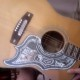### wordpress *won’t* let me publish my copy! quitting forever again soon i bet! dammit!!!!

number theory and physics.]
********************************************
Define f(n) = n – \phi(n).
Then f is the function that, from
CRS(n)={0,1,…,n-1}
(a Complete Residue System (mod n)),
counts the elements “a”
such that (a,n) \not= 1.

We can think of this as counting
the objects of the set
ZD(n):= CRS(n) \setdifference RRS(n):
f(n) = #ZD(n).
(Objects of ZD(n) are sometimes called
“zero divisors” (mod n).)

Now if x \in ZD(d), we have (x,d) \not= 1
and so (recall d|n) we get (x,n) \not= 1 as well:
x \in ZD(n). Thus ZD(d) \subset ZD(n).
But then f(d) \le f(n),
or, in other words,
n – \phi(n) \ge d – \phi(d).

This is *almost* what we want…
the “\ge” (“is greater than or equal to”)
must be “made strict”… we must
*eliminate* the possibility that
the two sides of our equation are equal.

So notice that
d \not== 0 (mod n)
whereas
d == 1 (mod d).

Thus  and [d] represent
*different* objects (equivalence classes)
in ZD(n) but represent the *same* object
in ZD(d). It follows that the “\subset”
relation found three paragraphs above
is *strict*; our result follows:
n – \phi(n) > d – \phi(d).

1.vlorbik

PS

(If d|n and 0<d d – \phi(d).
)
[Let “\phi” denote “Euler’s Phi-function”
(the “totient” function)
defined by
\phi(n) = #RRS(n).
We’ve seen that \phi
“counts numbers prime to n”…
or, the same thing, counts elements
of a Reduced Residue System (mod n)
(these are the a \in CRS(n) such that
(a,n) = 1.)
]

Let d|n with 0<d d – \phi(d).

•vlorbik

right at the end
there’s some secret code
(hidden from my eyes) that causes
some evil software-demon to throw
away what should *follow* from here
(namely the rest of the post as written;
the bit that appears at the top level
of this blog *as* a post).

this used to be so *easy*!

2.vlorbik

original title: “that’s how cyclic groups roll”.

3.vlorbik

error patched moments ago.
after, like, a whole day. what,
no bug reports?

4.vlorbik

Click to access BSwDtalk.pdf

sinnott on swinnerton-dyer (slides from a talk…
unused there because of “technical difficulties”.

• ## (Partial) Contents Page

Vlorbik On Math Ed ('07—'09)
(a good place to start!)